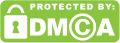Search for:

This post may contain affiliate links. If you click an affiliate link and make a purchase, I may earn a commission. Also, as an Amazon Associate I earn from qualifying purchases.

Pergola and Flagstone Patio Design
Pergola and Flagstone Patio Design

Pea gravel is small, rounded gravel that is suitable for use on walkways and driveways. This calculator helps give an estimate of the amount required to complete a project using this material.

To find out how many litres of pea gravel is needed to cover a specific area, follow these steps:

## Free Pea Gravel Calculator

Pea gravel is one of many choices when it comes to paving your driveway or walkway. This guide will show you the different options and calculations needed for pea gravel.

1) Measure the width and length of your surface in metres. For example, if the width is 2m and the length 5m, then your surface would be 10 square metres. Enter those figures here:

2) Find out what size (diameter) you require your pea gravel to be and enter it into the “Size” box below. Generally, we’d recommend 1/4″ (6mm), but 1/8″ (3mm) will also work. Enter the size of your required pea gravel:

3) If you want to know how much 1 cubic metre of material weighs then enter that figure here:

4) Press the calculate button and we’ll tell you how many litres of pea gravel you need:

[yarpp]

5) Finally, we’ll give you some price information. We won’t include freight or delivery costs because these vary too much depending on where you live. Instead, we list the cost per unit (i.e., per litre).

Based on the measurements you provide, you can work out the area and volume of the required pea gravel using the formulae detailed below:

Area=Length×Width

Volume=Area×Depth

Weight=Density×Volume

Best of all…

Our calculator can work out the total cost if you know the price per unit mass/volume:

Cost=Priceperunitmass×Weight

Cost=Priceperunitvolume×Volume

What if I don’t know the price per unit mass/volume?

You can work out these values from a bag of your purchased pea gravel, using the formulae:

Priceperunitmass=TotalcostTotalweightPriceperunitmass=TotalcostTotalweight

Priceperunitvolume=TotalcostTotalvolumePriceperunitvolume=TotalcostTotalvolume

Confused? 🤔

Below we show you three examples, detailing how to work out the volume, weight and cost.

Otherwise, simply enter your measurements for your landscaping project!

## Example calculations

• Pea Gravel Path

My path measures 20 feet by 30 feet wide.

I want coverage to a depth of 3 inches with normal pea gravel (96 lb/ft³) costing \$45 per US short ton.

I simply enter these measurements to do the following operations:

Area=Length×Width=200ft×300ft=600ft2Area=Length×Width=200ft×300ft=600ft2

Volume=Area×Depth=600ft2×3in=5.556yd3Volume=Area×Depth=600ft2×3in=5.556yd3

Weight=Density×Volume=96lb/ft3×5.556yd3=14,400lbWeight=Density×Volume=96lb/ft3×5.556yd3=14,400lb

Cost=Priceperunitmass×Weight=45\$/t×14,400lb=\$324Cost=Priceperunitmass×Weight=45\$/t×14,400lb=\$324

• Cover 0.1 Cubic Yards of Driveway Calculating Price Per Unit Volume

I can purchase 10 cubic feet for a cost of \$300 and I want 0.1 cubic yards for my path.

Priceperunitvolume=TotalcostTotalvolume=\$30010ft3=30\$/ft3Priceperunitvolume=TotalcostTotalvolume=\$30010ft3=30\$/ft3

I then calculate:

Weight=Density×Volume=96lb/ft3×0,1yd3=0,31tWeight=Density×Volume=96lb/ft3×0,1yd3=0,31t

Cost=Priceperunitvolume×Volume=30\$/ft3×0,31t=\$81Cost=Priceperunitvolume×Volume=30\$/ft3×0,31t=\$81

At this point, you may be asking:

What if I don’t know the density of the material?

You can calculate the density using the formula below and enter your value in the custom field:

Density=MassVolumeDensity=MassVolume

• Custom Aggregate Example

I want to cover 10 ft² of the path to a depth of 5 in with gravel weighing 200 lb per 4 cubic feet and costing \$45 for each US short ton.

Density=MassVolume=200lb4ft3=50lb/ft3Density=MassVolume=200lb4ft3=50lb/ft3

Volume=Area×Depth=10ft2×5in=0.154yd3Volume=Area×Depth=10ft2×5in=0.154yd3

Weight=Density×Volume=50lb/ft3×0.154yd3=0.104tWeight=Density×Volume=50lb/ft3×0.154yd3=0.104t

Cost=Priceperunitmass×Weight=45\$/t×0.104t=\$4.69Cost=Priceperunitmass×Weight=45\$/t×0.104t=\$4.69

At this point, it’s likely that you are asking the following question:

## What if my measurements are in different units?

You might notice that we changed between units (e.g. Density is in pounds per cubic feet and volume is given in cubic yards).

The calculator does the following conversions for you:

1ft=12in=0.33yd=30.48cm=0.3048m1ft=12in=0.33yd=30.48cm=0.3048m

1t=2000lb=0.893longt=907kg1t=2000lb=0.893longt=907kg

We think that our pea gravel calculator is a powerful online tool that can help you find out how many bags of gravel you need, eliminate wasted stones, and of course save you money!We own and operate multiple Outdoor and Gardening sites. Its our passion to show our readers all the amazing things they can do to their patios and backyard. There is no bigger pleasure for us then to share with you our readers our experience in Gardening , Landscaping and Decorating.Pin It

## GET 50% Off From Amazon From Our Patio Store

* indicates required

No, thank you. I do not want.
100% secure your website.